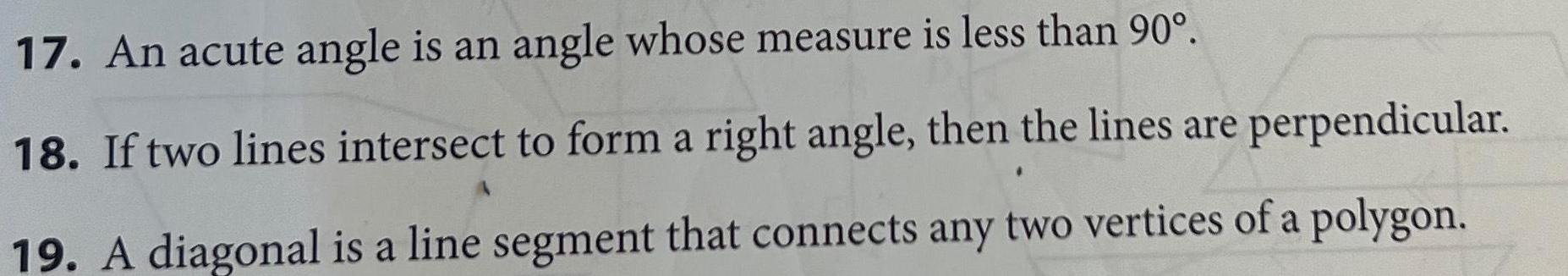Question:

# 17 An acute angle is an angle whose measure is less than 90

Last updated: 9/15/202317 An acute angle is an angle whose measure is less than 90 18 If two lines intersect to form a right angle then the lines are perpendicular 19 A diagonal is a line segment that connects any two vertices of a polygon